# Problem 6. (Mean Value Property) Let f : RR be a function with continuous second derivative. (a) Suppose f"( to f(...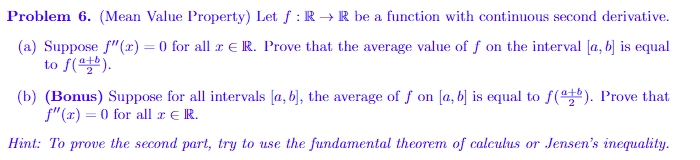Problem 6. (Mean Value Property) Let f : RR be a function with continuous second derivative. (a) Suppose f"( to f( ). 0 for all r E IR. P al rove that the average value of f on the interval a, bs equ f, onla b is equal tore !) Prove intervals la, b, the average。 (b) (Braus) Supposeerall Hint: To prove the second part, try to use the fundamental theorem of calculus or Jensen's inequality.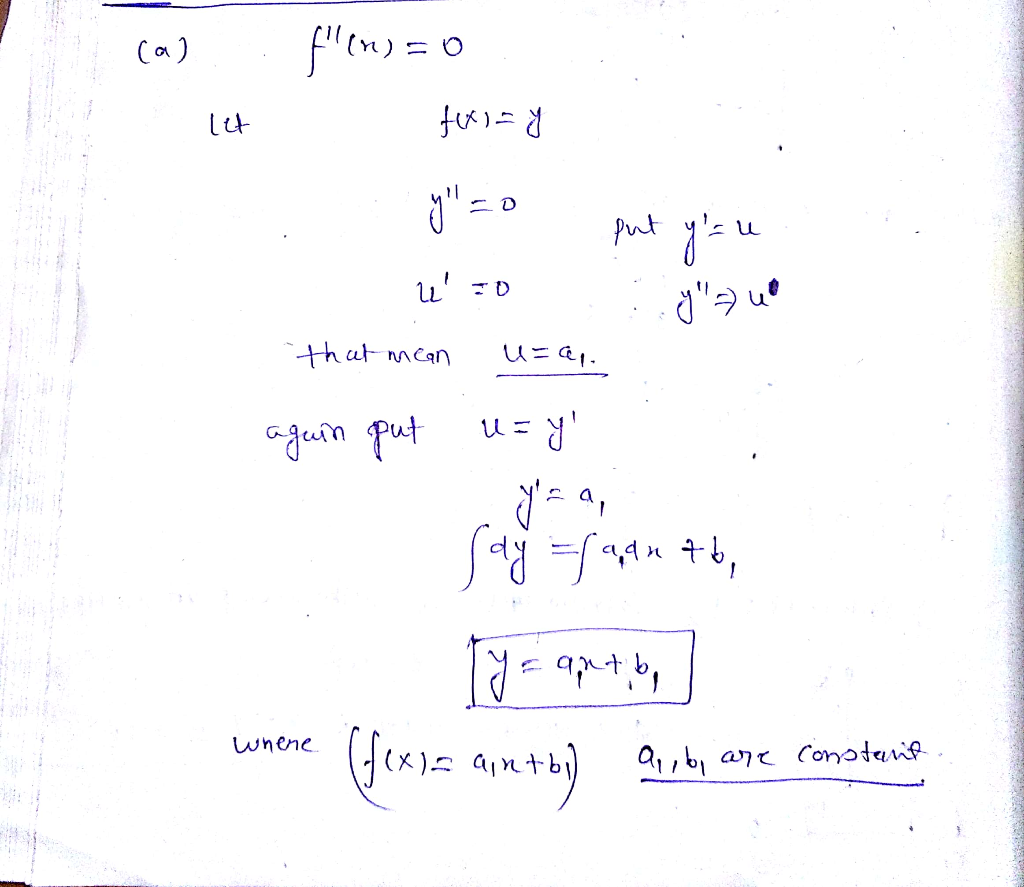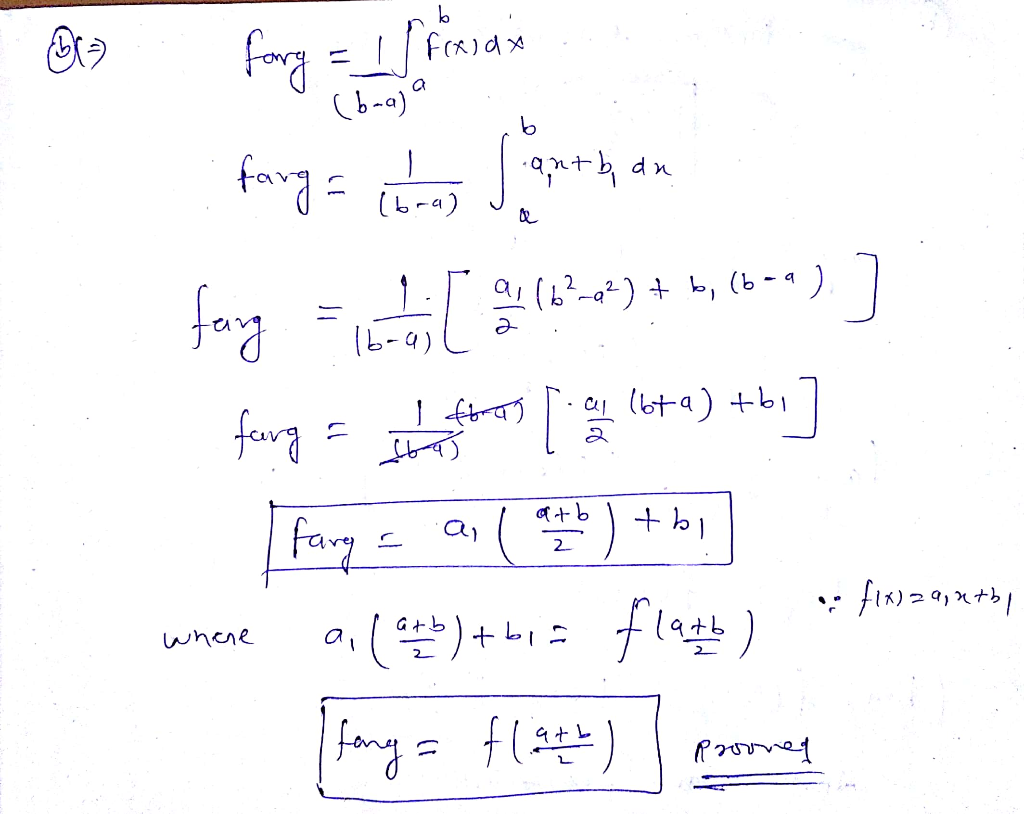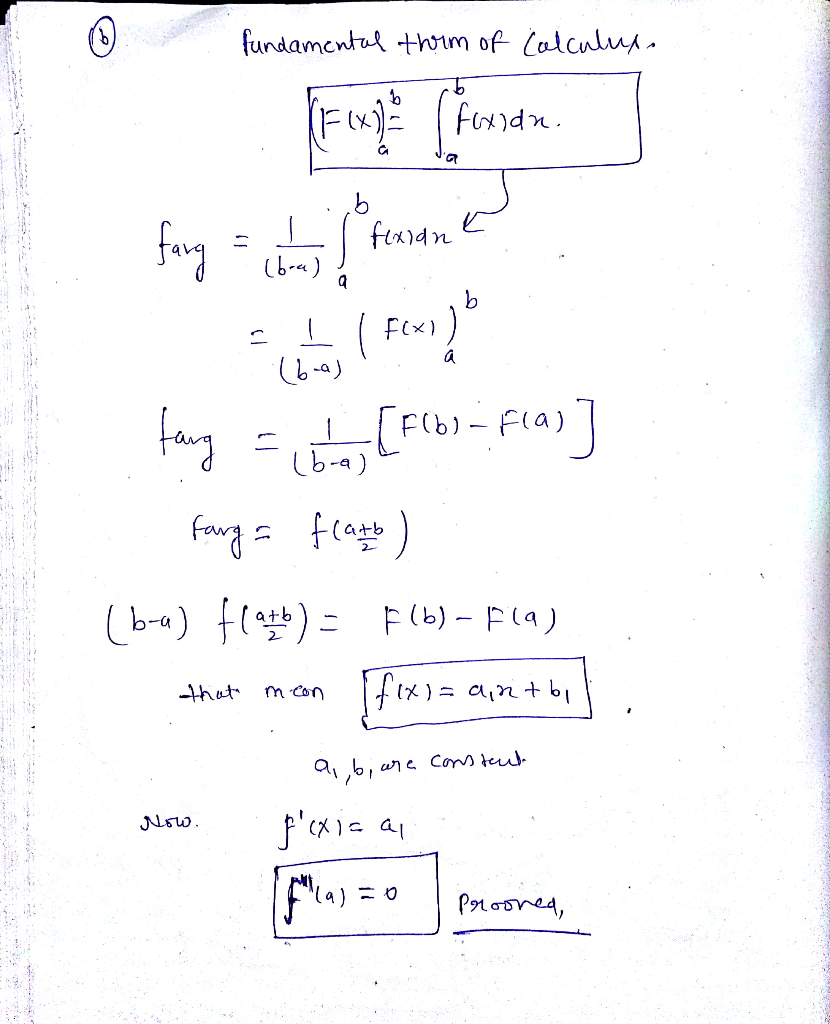##### Add Answer of: Problem 6. (Mean Value Property) Let f : RR be a function with continuous second derivative. (a) Suppose f"( to f(...
Similar Homework Help Questions
• ### Problem 6. (Mean Value Property) Let f : RR be a function with continuous second derivative. (a) Suppose f"( to f(...Problem 6. (Mean Value Property) Let f : RR be a function with continuous second derivative. (a) Suppose f"( to f( ). 0 for all r E IR. P al rove that the average value of f on the interval a, bs equ f, onla b is equal tore !) Prove intervals la, b, the average。 (b) (Braus) Supposeerall Hint: To prove the second part, try to use the fundamental theorem of calculus or Jensen's inequality. Problem 6. (Mean Value...

• ### Theorem 20.8 (The Mean Value Theorem for Integral Calculus). Let f a, bR be continuous, and g a, ...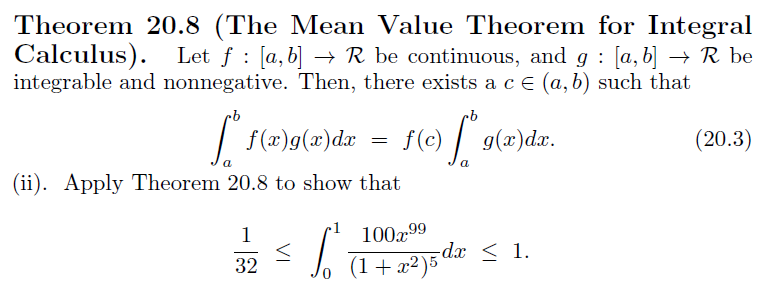Theorem 20.8 (The Mean Value Theorem for Integral Calculus). Let f a, bR be continuous, and g a, bR be integrable and nonnegative. Then, there exists acE (a,b) such that (20.3) f(x)g(a)dx - f(c g(x)dr (ii). Apply Theorem 20.8 to show that 1 100 32 Jo (1 +r2)5 Theorem 20.8 (The Mean Value Theorem for Integral Calculus). Let f a, bR be continuous, and g a, bR be integrable and nonnegative. Then, there exists acE (a,b) such that (20.3) f(x)g(a)dx...

• ### 2. Let f: R R be a continuous function. Suppose that f is differentiable on R\{0} and that there exists an L e R such that lim,of,(z) = L. Prove that f is differentiable at 1-0 with f,(0) = L. (Hint:...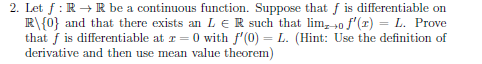2. Let f: R R be a continuous function. Suppose that f is differentiable on R\{0} and that there exists an L e R such that lim,of,(z) = L. Prove that f is differentiable at 1-0 with f,(0) = L. (Hint: Use the definition of derivative and then use mean value theorem) 2. Let f: R R be a continuous function. Suppose that f is differentiable on R\{0} and that there exists an L e R such that lim,of,(z) =...

• ### (8) 2 points Let f be a function defined and continuous, with continuous first partial derivative at the origin (0,...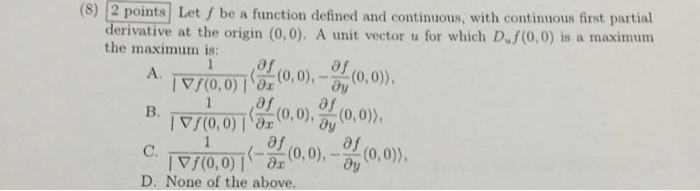(8) 2 points Let f be a function defined and continuous, with continuous first partial derivative at the origin (0,0). A unit vector u for which D.f (0,0) is the maximum is: maximum a 1 (0,0)), A. /(0,0)x,0),y (0 af B. (0,0) 8x0,0),(0,0)), af 1 ((0,0),-y C. (0,0), /(0,0) D. None of the above. (8) 2 points Let f be a function defined and continuous, with continuous first partial derivative at the origin (0,0). A unit vector u for which...

• ### Let f(x) be a function on Rn with continuous first-order partial derivatives and let M be a subsp...

Let f(x) be a function on Rn with continuous first-order partial derivatives and let M be a subspace of Rn. Assume that x* ∈ M. Suppose x* minimizes f(x) on M. Prove that ∇f(x*) ∈ M⊥ Assume, in addition, that f(x) is convex and ∇f(x*) ∈ M⊥. Prove that x* is a global minimizer of f(x) on M.

• ### Let s < t and let f:[s,t]→ℝ be a differentiable function. Suppose that f'(x) > 0 for all x ...

Let s < t and let f:[s,t]→ℝ be a differentiable function. Suppose that f'(x) > 0 for all x Which of the following is correct? 1. Using the definition of the derivative, it follows that f(x)<f(a) for any x<a. 2. Using Rolle's Theorem, it follows that f is a continuous function. 3. Using the Mean Value Theorem, it follows that f(t)>f(s). 4. Using Rolle's Theorem, it follows that there is some x∈[s,t] such that f′(x)=0. 5. Using the Intermediate Value...

• ### (a) Suppose that f is continuous on [0, 1] and f(o) = f(1). Let n be 20. any natural number. Prov...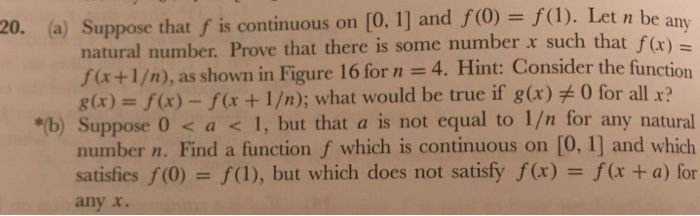(a) Suppose that f is continuous on [0, 1] and f(o) = f(1). Let n be 20. any natural number. Prove that there is some number x such that f fx+1/m), as shown in Figure 16 for n 4. Hint: Consider the function g(x) = f(x)-f(x + 1/n); what would be true if g(x)ヂ0 for all x? "(b) Suppose 0 < a 1, but that a is not equal to 1/n for any natural number n. Find a function f...

• ### 1. (25 pts) Let f(x) be a continuous function and suppose we are already given the Matlab function "f.", with header "function y fx)", that returns values of f(x) Given the following...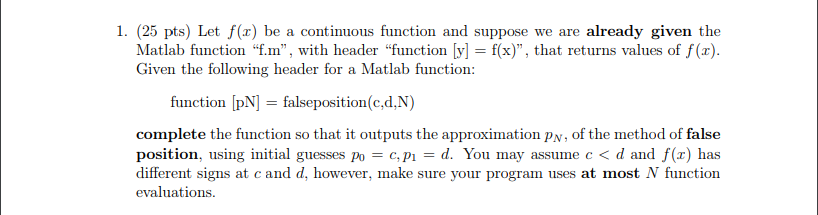1. (25 pts) Let f(x) be a continuous function and suppose we are already given the Matlab function "f.", with header "function y fx)", that returns values of f(x) Given the following header for a Matlab function: function [pN] falseposition(c,d,N) complete the function so that it outputs the approximation pN, of the method of false position, using initial guesses po c,pd. You may assume c<d and f(x) has different signs at c and d, however, make sure your program uses...

• ### Problem 2 (3 points) Suppose that is increasing in a,b]. Let zo є [a,b] such that is continuous at xo. Given a function f on [a,b] such that f(m) = 1 and f(x) 0 elsewhere prove that f eR(a) and that...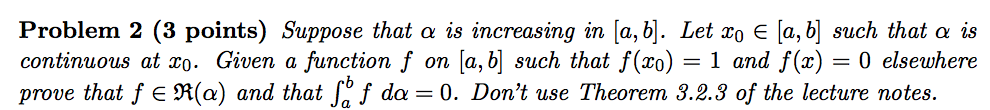Problem 2 (3 points) Suppose that is increasing in a,b]. Let zo є [a,b] such that is continuous at xo. Given a function f on [a,b] such that f(m) = 1 and f(x) 0 elsewhere prove that f eR(a) and that Jo f da -0. Don't use Theorem 3.2.3 of the lecture notes. Problem 2 (3 points) Suppose that is increasing in a,b]. Let zo є [a,b] such that is continuous at xo. Given a function f on [a,b] such...

• ### 1 Let f (t), g(t) be a continuous function on some interval I, and to e I. Prove that the initial value problem y'(t) f(t)y + g(t)y2, y(to) zo has a unique and continuous solution φ(t) on a s...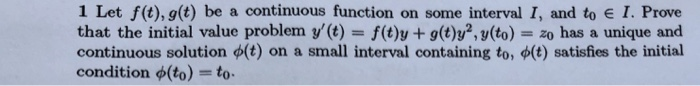1 Let f (t), g(t) be a continuous function on some interval I, and to e I. Prove that the initial value problem y'(t) f(t)y + g(t)y2, y(to) zo has a unique and continuous solution φ(t) on a small interval containing to, φ(t) satisfies the initial condition φ(to) = to. 1 Let f (t), g(t) be a continuous function on some interval I, and to e I. Prove that the initial value problem y'(t) f(t)y + g(t)y2, y(to) zo has...

Need Online Homework Help?